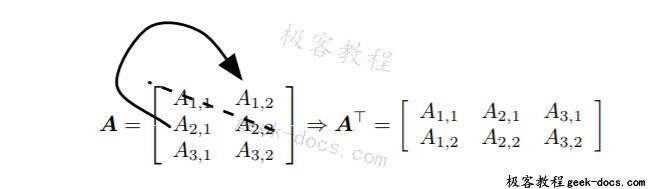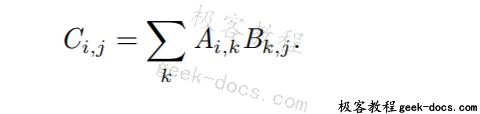# 矩阵和向量相乘A^T i,_j = A矩阵和向量相乘

（matrix product）是第三个矩阵 C。为了使乘法定义良好，矩阵 A 的列数必须和矩阵 B 的行数相等。如果矩阵 A 的形状是 m × n，矩阵 B 的形状是 n × p，那么矩阵C 的形状是 m × p。我们可以通过将两个或多个矩阵并列放置以书写矩阵乘法，例如C = AB. 具体地，该乘法操作定义为x^⊤y = y^⊤x.

(AB)^⊤ = B^⊤ A^⊤

x^⊤y =(x⊤y)^⊤ = y^⊤ x.

• 回顶